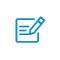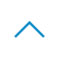•• 注册 忘记密码?

# 用于可解释机器学习的 Python 库# yellowbrick

1. pip install yellowbrick

1. import pandas as pd
2.
3. from sklearn import datasets
4.
5. wine_data = datasets.load_wine()
6.
7. df_wine = pd.DataFrame(wine_data.data,columns=wine_data.feature_names)
8.
9. df_wine['target'] = pd.Series(wine_data.target)

1. import pandas as pd
2.
3. from sklearn import datasets
4.
5. wine_data = datasets.load_wine()
6.
7. df_wine = pd.DataFrame(wine_data.data,columns=wine_data.feature_names)
8.
9. df_wine['target'] = pd.Series(wine_data.target)

1. import pandas as pd
2.
3. from sklearn import datasets
4.
5. wine_data = datasets.load_wine()
6.
7. df_wine = pd.DataFrame(wine_data.data,columns=wine_data.feature_names)
8.
9. df_wine['target'] = pd.Series(wine_data.target)1. from yellowbrick.classifier import ClassificationReport
2.
3. from sklearn.ensemble import RandomForestClassifier
4.
5. model =  RandomForestClassifier()
6.
7. visualizer = ClassificationReport(model, size=(1080720))
8.
9. visualizer.fit(X_train, y_train)
10.
11. visualizer.score(X_test, y_test)
12.
13. visualizer.poof()## ELI5

ELI5是另一个可视化工具包，在模型机器学习模型调试和解释其产出的预测结果方面非常有用。它能够同大多数通用的python机器学习工具包一起使用，包括scikit-learn和XGBoost，以及Keras。

1. import eli5
2.
3. eli5.show_weights(model, feature_names = X.columns.tolist())

1. from eli5 import show_predictionshow_prediction(model, X_train.iloc, feature_names = X.columns.tolist(),
2.
3.                 show_feature_values=True)## LIME

LIME（模型无关局部可解释）是一个用来解释模型做出的预测的工具包。LIME支持对多种分类器的单个样本预测进行解释，并且原生支持scikit-learn。

LIME可以用pip进行安装

1. pip install lime

1. import lime.lime_tabular
2.
3. explainer = lime.lime_tabular.LimeTabularExplainer(X_train.values,                                            feature_names=X_train.columns.values.tolist(),                                        class_names=y_train.unique())

1. predict_fn = lambda x: model.predict_proba(x).astype(float

1. exp = explainer.explain_instance(X_test.values, predict_fn, num_features=6
2.
3. exp.show_in_notebook(show_all=False)# MLxtend

1. pip install mlxtend

1. from mlxtend.plotting import plot_decision_regions
2.
3. from mlxtend.classifier import EnsembleVoteClassifier
4.
5. import matplotlib.gridspec as gridspec
6.
7. import itertools
8.
9. from sklearn import model_selection
10.
11. from sklearn.linear_model import LogisticRegression
12.
13. from sklearn.naive_bayes import GaussianNB
14.
15. from sklearn.ensemble import RandomForestClassifier

1. X_train_ml = X_train[['proline''color_intensity']].values
2.
3. y_train_ml = y_train.values

1. clf1 = LogisticRegression(random_state=1
2.
3. clf2 = RandomForestClassifier(random_state=1
4.
5. clf3 = GaussianNB()
6.
7. eclf = EnsembleVoteClassifier(clfs=[clf1, clf2, clf3], weights=[1,1,1])
8.
9. value=1.5
10.
11. width=0.75
12.
13. gs = gridspec.GridSpec(2,2
14.
15. fig = plt.figure(figsize=(10,8))
16.
17. labels = ['Logistic Regression''Random Forest''Naive Bayes''Ensemble'
18.
19. for clf, lab, grd in zip([clf1, clf2, clf3, eclf],
20.
21.                          labels,
22.
23.                          itertools.product(, repeat=2)):
24.
25.
26.
27.     clf.fit(X_train_ml, y_train_ml)
28.
29.     ax = plt.subplot(gs[grd, grd])
30.
31.     fig = plot_decision_regions(X=X_train_ml, y=y_train_ml, clf=clf)
32.
33.     plt.title(lab)Copyright©2005-2020 Sykv.com 可思数据 版权所有    京ICP备14056871号-1扫码入群返回顶部